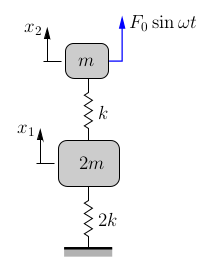### Multiple Degree of Freedom Systems: Chapter 8 Examples

### Example 8-1

The double pulley shown below (which is supported by a spring with stiffness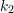) has a moment of inertia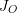about its center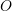. The pulley supports a mass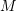by a light cable which has an effective stiffness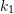. The coordinatesand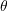as shown are used to describe the system.1. Determine the equations of motion for this system using Newton’s Law’s.
2. If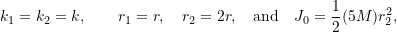the equations of motion become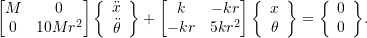Determine the resulting natural frequencies for this system and sketch the associated mode shapes.

### Example 8-2

Determine expressions for the natural frequencies and mode shapes for the system shown. Use the coordinates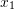and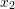as indicated.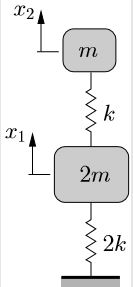### Example 8-3

Two simple pendulums of the same length are connected by a light spring with stiffness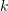as shown.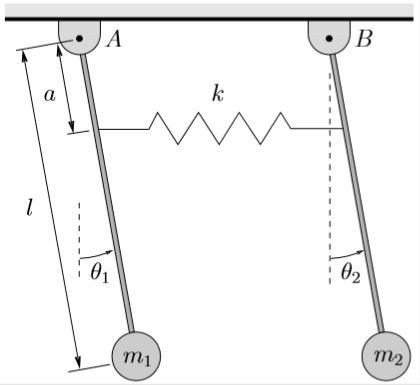For this system, determine

1. the equations of motion,
2. the natural frequencies,
3. the mode shapes,
4. the total response of the system (for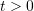) if the initial conditions at time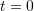are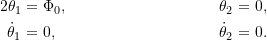For parts (b)–(d) assume that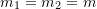.

### Example 8-4

A rigid beam (mass, length, centroidal moment of inertia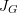) is supported by springs at its ends as shown. Determine the equations of motion, natural frequencies and mode shapes for this system.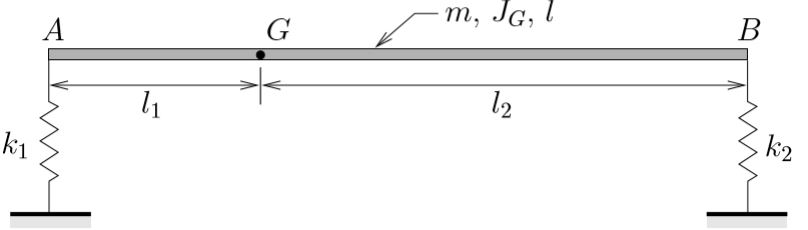Solve this problem using two different sets of coordinates:

1.and,
2.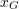and,

as illustrated below.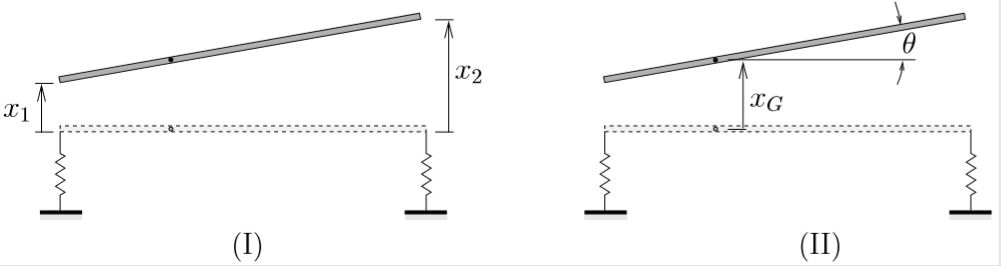Be sure to identify any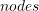in each mode shape.

### Example 8-5

An asymmetric machine mounting is supported by both vertical and horizontal springs as shown below.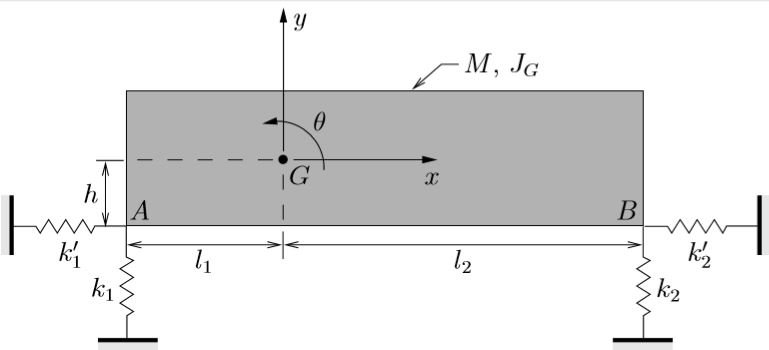1. Using the coordinates,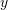, andas illustrated, determine the equations of motion for this system.
2. Explain how the equations of motion and mode shapes change in each of the following special cases:
1.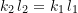,
2.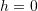,
3.and.

### Example 8-6

The two degree of freedom system shown consists of a wheel (with mass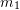and centroidal moment of inertia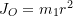) which rolls without slipping and a bar (with mass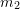and centroidal moment of inertia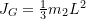) connected by three springs each of stiffness.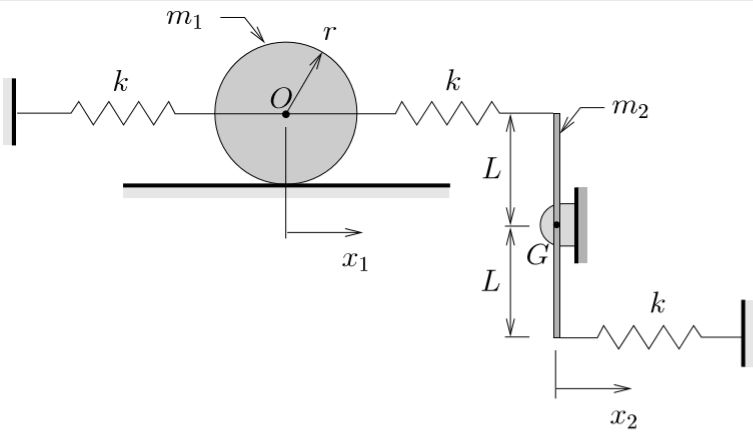1. Using the coordinates(the horizontal position of the center of the wheel) and(the horizontal position of the lower end of the bar) show that if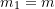and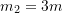, the equations of motion for this system become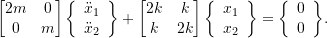2. Determine the natural frequencies and mode shapes for this system.

### Example 8-7

Determine the steady state response for the system shown.